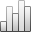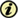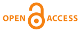More Statisticssince 05 February 2011 :
View(s): 400 (0 ULiège)

### Solving Graph Bandwidth Minimization Problem Using Imperialist Competitive Algorithm

Article#### Attached document(s)

Version PDF originale

#### Abstract

The bandwidth minimization problem can be used in data storage and VLSI design issues and saving large hypertext media, etc. The Matrix Bandwidth Minimization Problem involves finding matrix rows and columns permutation so that non-zero elements of the matrix A are located in a band that is as close as possible to the original diameter to minimize the amount of {max{|i−j|:aij≠.}. The Bandwidth Minimization Problem for Graphs (BMPG) is a complicated problem; hence the deterministic algorithms are not appropriate to solve these kinds of problems. The purpose of this research is to reduce the required computations through the use of heuristic algorithms and evolutionary algorithms, so that instead of using purely mathematical methods to find answers, we can turn the problem into an optimization problem through the use of collective intelligence and evolutionary algorithms and the concepts in this field. In the present paper, the use of meta-heuristic algorithm, Imperialist competitive algorithm is proposed in order to solve minimization problem. In this paper, the performance of presented algorithm with random samples has been evaluated compared with the results of genetic algorithms. The results of tests show that the Imperialist competitive algorithm can be considered as an efficient method to solve the bandwidth minimization problem for graphs.

Keywords : genetic algorithm, graph bandwidth, imperialist competitive algorithm, matrix bandwidth

Amir Aliabadian, Mohammad-Rasol Jafari & Ali Azarbad, «Solving Graph Bandwidth Minimization Problem Using Imperialist Competitive Algorithm», Bulletin de la Société Royale des Sciences de Liège [En ligne], Volume 86 - Année 2017, Special issue, 493 - 508 URL : https://popups.uliege.be:443/0037-9565/index.php?id=6844.